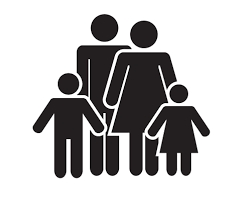# Represent 7494

There are 12 more than boys, and the boys represent 30% of all pupils in the class. Find how many girls are in the class.

d =  21

### Step-by-step explanation:

d = 12 + c
c = 0.30 · (c+d)

c-d = -12
0.7c-0.3d = 0

Row 2 - 0.7 · Row 1 → Row 2
c-d = -12
0.4d = 8.4

d = 8.4/0.4 = 21
c = -12+d = -12+21 = 9

c = 9
d = 21

Our linear equations calculator calculates it.Did you find an error or inaccuracy? Feel free to write us. Thank you!

Tips for related online calculators
Need help calculating sum, simplifying, or multiplying fractions? Try our fraction calculator.
Do you have a system of equations and looking for calculator system of linear equations?